• 只是python代码方便以后调用，理论部分需要看其他人的回归模型的预测import matplotlibimport matplotlib.pyplot as pltimport pandas as pdimport numpy as npimport seaborn as snsimport statsmodels.api as sm...

只是python代码方便以后调用，理论部分需要看其他人的

回归模型的预测

import matplotlib

import matplotlib.pyplot as plt

import pandas as pd

import numpy as np

import seaborn as sns

import statsmodels.api as sm

from sklearn import model_selection

from scipy.stats import f

from scipy.stats import norm

font = {

'family': 'FangSong',

'weight': 'bold',

'size': 12

}

matplotlib.rc("font", **font)

Profit = pd.read_excel("../data/Predict to Profit.xlsx", names=list("abcde"))

'''

RD_Spend 49 non-null float64

Marketing_Spend 49 non-null float64

State 49 non-null object

Profit 49 non-null float64

'''

print(Profit.shape)

# 将数据拆分成训练集和测试集

train, test = model_selection.train_test_split(Profit, test_size=0.2, random_state=1234)

# 根据train数据集建模

model = sm.formula.ols('e ~ a+b+c+C(d)', data=train).fit()

# 删除test集中的Profit变量，用剩下的自变量进行预测

test_X = test.drop(labels='e', axis=1)

pred = model.predict(exog=test_X)

# 对比预测值和实际值的差异

print(pd.DataFrame({

'pred': pred,

'real': test.e

}))

模型的显著性检验 F检验# 计算建模数据中因变量的均值

ybar = train.e.mean()

# 统计变量个数和观测个数

p = model.df_model # 变量个数

n = train.shape # 观测个数

# 计算回归离差平方和

RSS = np.sum((model.fittedvalues - ybar) ** 2)

# 计算误差平方和

ESS = np.sum((train.e - model.fittedvalues) ** 2)

# 计算F统计量的值

F = (RSS/p)/(ESS/(n - p - 1))

# 直接得到F统计量值

F1 = model.fvalue

print(F)

# 对比结果下结论

# 计算F分布的理论值

F_Theroy = f.ppf(q=0.95, dfn=p, dfd=n-p-1)

print(F_Theroy)

回归系数的显著性检验 t检验

print(model.summary())P>|t|的值小于0.05才有用

回归模型的诊断

①误差项ε服从正态分布

误差项服从正太分布，就是要求因变量服从正态分布

绘制直方图

sns.distplot(a=Profit.e, bins=10, norm_hist=True, fit=norm,

hist_kws={'color': 'steelblue'},

kde_kws={'color': 'black', 'linestyle': '--', 'label': '核密度图'},

fit_kws={'color': 'red', 'linestyle': ':', 'label': '正态密度曲线'})

plt.legend()

# 显示图形

plt.show()②无多重共线性

关于多重共线性的检验可以使用方差膨胀因子VIF来鉴定，如果VIF大于10，则说明变量间存在多重共线性；如果VIF大于100,则表名变量间存在严重的多重共线性如果发现变量之间存在多重共线性的话，则可以考虑删除变量或重新选择模型

# 导入statsmodel模块函数

from statsmodels.stats.outliers_influence import variance_inflation_factor

# 自变量X(包含RD_Speed、Marketing_Speed和常数列1)

# 构造空的数据框，用于存储VIF值

vif = pd.DataFrame()

vif['features'] = X.columns

vif['VIF Factor'] = [variance_inflation_factor(X.values, i) for i in range(X.shape)]

print(vif)③线性相关性

高度相关:|p| >= 0.8

中度相关:0.5 <= |p| <0.8

弱相关:0.3 <= |p| < 0.5

几乎不相关:|p| < 0.3

相关性越大越好

# 计算数据集Profit中每个自变量与因变量利润之间的相关系数

res = Profit.drop(labels=['e'], axis=1).corrwith(Profit.e)

print(res)

# 绘制散点图矩阵

sns.pairplot(Profit.loc[:, ['a', 'b', 'c', 'e']])

plt.show()综合考虑相关系数、散点图矩阵和t检验的结果,最终确定只保留模型model中的a(RD_Speed)和c(Marketing_Speed)两个自变量重新对该模型做修正

model2 = sm.formula.ols('e ~ a + c', data=train).fit()

# 模型回归系数的估计值 e = 51902.112471 + 0.79*a + 0.02*c

print(model2.params)

异常值检验

通常利用帽子矩阵、DFFITS准则、学生化残差或Cook距离进行异常点检测

outliers = model2.get_influence()

# 高杠杆值点(帽子矩阵)

leverage = outliers.hat_matrix_diag

# diffits值

dffits = outliers.dffits

# 学生化残差

resid_stu = outliers.resid_studentized_external

# cook距离

cook = outliers.cooks_distance

# 合并各种异常值检验的统计量值

contat1 = pd.concat([pd.Series(leverage, name='leverage'), pd.Series(dffits, name='dffits'),

pd.Series(resid_stu, name='resid_stu'), pd.Series(cook, name='cook')], axis=1)

# 重设train数据的行索引

train.index = range(train.shape)

# 将上面的统计量与train数据集合并

profit_outliers = pd.concat([train, contat1], axis=1)

# 为了简单起见，这里使用标准化残差，当标准化残差大于2时，即认为对应的数据点为异常点

outliers_ratio = sum(np.where((np.abs(profit_outliers.resid_stu) > 2), 1, 0))/profit_outliers.shape

print(outliers_ratio)

# 异常比例不高，低于5%，可以考虑删除

# 挑选非异常观测点

none_outliers = profit_outliers.loc[np.abs(profit_outliers.resid_stu) <= 2, :]

# 应用无异常值的数据集重新建模

model3 = sm.formula.ols('e ~ a + c', data=none_outliers).fit()

print(model3.params)

方差齐性检验

方差齐性是要求模型残差项的方差不随自变量的变动而呈现某种趋势，否则，残差的趋势就可以被自变量刻画。

# 设置第一张子图的位置

ax1 = plt.subplot2grid(shape=(2, 1), loc=(0, 0))

# 绘制散点图

ax1.scatter(none_outliers.a, (model3.resid - model3.resid.mean())/model3.resid.std())

# 添加水平参考线

ax1.hlines(y=0, xmin=none_outliers.a.min(), xmax=none_outliers.a.max(), colors='red', linestyles='--')

# 添加x轴和y轴标签

ax1.set_xlabel('RD_Spend')

ax1.set_ylabel('Std_Residual')

# 设置第二张子图的位置

ax2 = plt.subplot2grid(shape=(2, 1), loc=(1, 0))

# 绘制散点图

ax2.scatter(none_outliers.c, (model3.resid - model3.resid.mean())/model3.resid.std())

# 添加水平参考线

ax2.hlines(y=0, xmin=none_outliers.c.min(), xmax=none_outliers.c.max(), colors='red', linestyles='--')

# 添加x轴和y轴标签

ax2.set_xlabel('Marketing_Spend')

ax2.set_ylabel('Std_Residual')

# 调整子图之间的水平间距和高度间距

# 显示图形

plt.show()

# 回归模型的预测

pred3 = model3.predict(exog=test.loc[:, ['a', 'c']])

# 绘制预测值与实际值的散点图

plt.scatter(x=test.e, y=pred3)

# 添加斜率为1、截距项为0的参考线

plt.plot([test.e.min(), test.e.max()], [test.e.min(), test.e.max()], color='red', linestyle='--')

# 添加轴标签

plt.xlabel('实际值')

plt.ylabel('预测值')

# 显示图形

plt.show()展开全文• “ Take a Bath” 是2008年美国大学生数学建模竞赛的Ａ 题。上次我们已经通过题目对冰盖...多元线性回归模型基于前面收集的数据和已有结果， 这里将建立多元线性回归模型来预测由于北极冰雪融化 (主要是格陵兰冰盖和...“ Take  a Bath” 是2008年美国大学生数学建模竞赛 的 Ａ 题。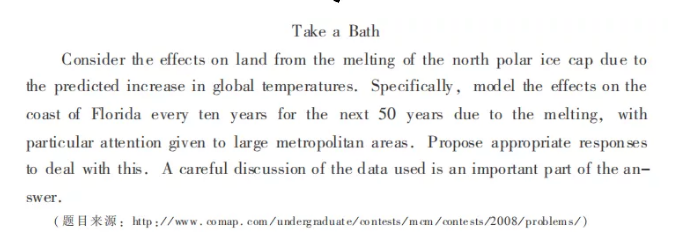上次我们已经通过题目对冰盖融化问题进行了详细分析并确立了基本模型

友情链接：

美赛A题解析之冰盖融化问题分析

美赛A题解析之冰盖融化问题模型预测

今天我们详细解读预测模型如何预测全球海平面上升幅度。

多元线性回归模型

基于前面收集的数据和已有结果， 这里将建立多元线性回归模型来预测由于北极冰雪融化 (主要是格陵兰冰盖和北极海冰的融化) 所导致的全球海平面上升幅度。如图 １－１６ 所示为使用多元线性回归模型对海平面上升幅度进行预测的流程。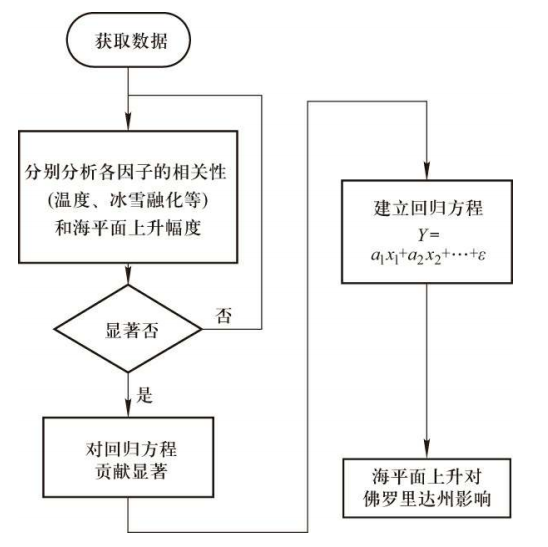图1-16

使用多元线性回归模型对海平面上升幅度进行预测的流程

众所周知， ＣＯ 等温室气体的排放以及冰雪消融对全球气温的升高具有重要影响。同时， 全球气温的升高和冰雪消融对海平面的升高也具有重要的影响。因此， 首先需要根据因素之间的相关性选取对海平面上升影响最显著的因素。

• 温室气体和全球气温变化之间的相关性。

• 北极冰雪融化和全球气温之间的相关性。

• 北极冰雪融化和海平面变化之间的相关性。

• 全球气温变化和海平面变化之间的相关性。相关性分析的结果见表 １－１ 。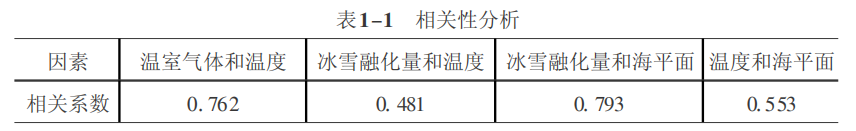显然， 全球气温直接受到温室气体排放和北极冰雪融化的影响。因此， 这里首先建立对全球温度变化进行预测的多元线性回归模型。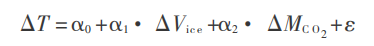式中， ΔVice 表示北极冰雪融化量， ΔMco2表示CO2的排放量。

全球海平面的上升将受到北极冰雪融化和全球气温变化的直接影响。类似地， 可以建立多元线性回归模型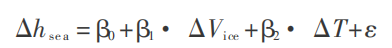为了确定上述多元线性回归模型的系数， 这里选取了佛罗里达州四个城市 (佛罗里达东海岸的圣彼得斯堡和彭萨科拉， 佛罗里达西海岸的费南迪纳比奇 和弗吉尼亚岛) 附近的海面的观测数据。回归方程 (1.67 ) 和 ( 1.17) 的系数可以使用spss软件确定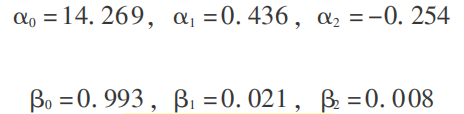显著性检验的结果表明， α１ 和 α２ 、 β１ 和 β２ 都是显著的， 而且线性关系也 比较显著———这也说明选择多元线性回归模型对全球平均气温和全球海平面上升幅度进行预测是比较合理的。

模型的求解和结果分析

根据收集的数据， 求解建立的模型，可以预测未来５０ 年中全球海平面的上升幅度、 佛罗里达附近海平面的上升幅度以及对海岸线的侵蚀。

( 一) 全球海平面的上升幅度

求解预测模型， 得到全球气温在未来 50年的变化预测值 ( 图1-17) 。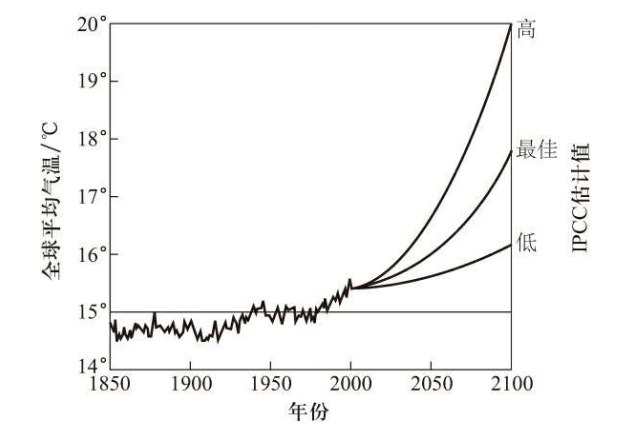图 １－１７　全球气温预测

IPCC报告中全球未来50年的温度预测见表 １－２ 。

表 1－2 IPCC 报告中全球未来 50 年的温度预测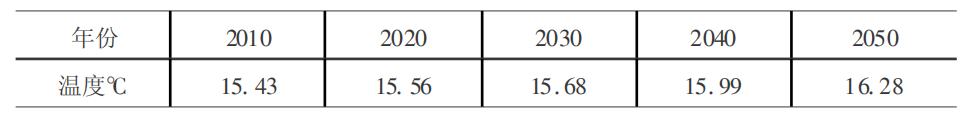使用预测模型 ( 1.17) 对未来 ５０ 年由于北极冰雪融化所导致的海平面上 升幅度进行了预测， 具体结果如图 １－１８ 所示。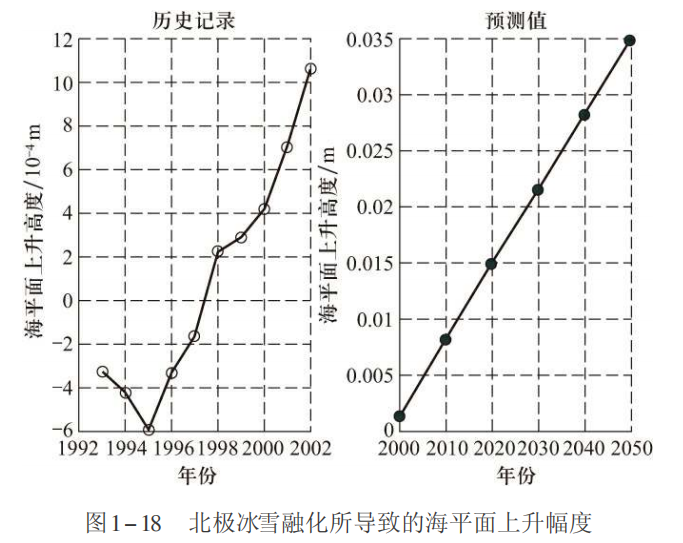图１－１８　北极冰雪融化所导致的海平面上升幅度

根据上面的计算结果不难发现， 在未来50年中， 北极冰雪融化将导致海 平面显著上升。

( 二) 佛罗里达附近海平面的上升幅度

根据题目的要求， 这里将预测佛罗里达州附近海平面的上升幅度， 仍然以费南迪 纳 比 奇 、 圣彼得斯堡  、 彭萨科拉和弗吉尼亚岛四个海岸城市为例。

因为Fernandina是最靠近Jacksonville的观测中心， 这里选取它的数据来确定回归模型的系数， 然后再进行预测。使用 ＳＰＳＳ 软件易得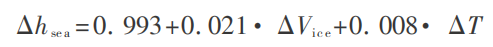根据该模型， Fernandina附近海平面高度见表1-3。

表 1－3 Fernandina 附近海平面高度／m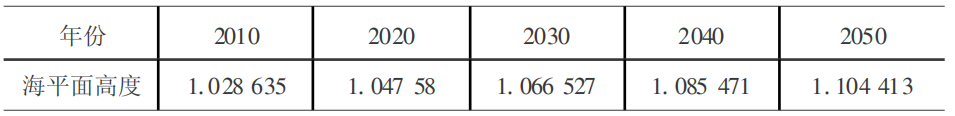根据该表不难发现， Ｆｅｒｎａｎｄｉｎａ 附近的海平面将以每年 ２ ｍｍ 的速度持续上升 (见图 １－１９) 。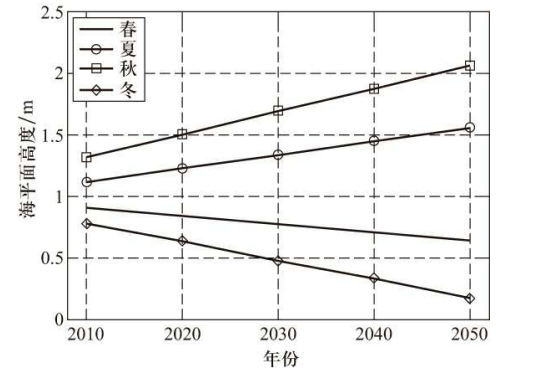图1-19

北极冰雪融化所导致的Fernandina附近海平面的上升幅度

同样的， 使用上述预测模型可以得到其他几个城 市的海平面上升幅度 (见表 １-４ 和图 1-20 ) 。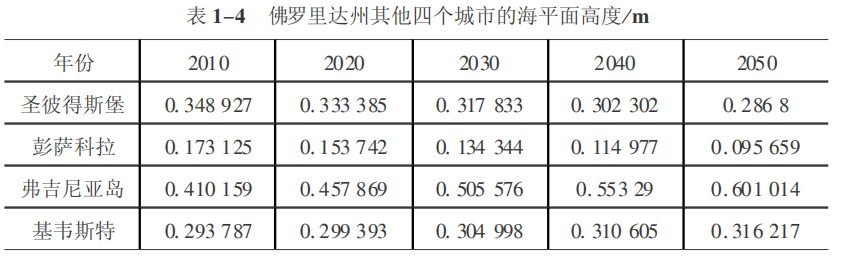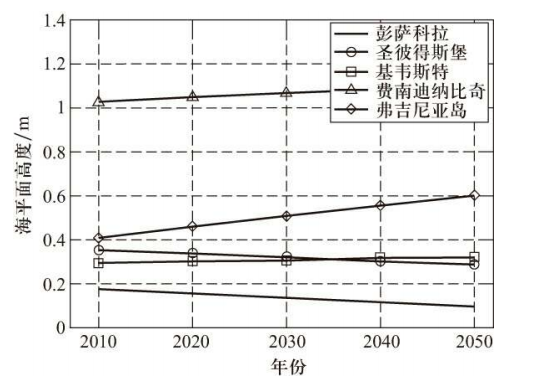图1-20　佛罗里达州５ 个大城市附近海平面的上升趋势图

( 三) 对海岸线的侵蚀

当海平面上升时， 它会产生两方面的影响。首先， 部分较低的陆地和河岸会被海水淹没、 侵占， 因此， 海岸线会向内陆侵蚀。但是， 通过多年的气象观测可以证明这种影响是非常弱的。其次， 随着海平面上升， 海洋的波浪作用也 可能对海岸造成巨大的额外侵蚀。根据布容法则 ( Ｂｒｕｕ ｎ Ｒｕｌｅ)可以计算出被侵蚀的海岸线距离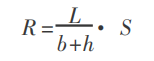式中， R 表示海岸线被侵蚀的距离， b 表示海边沙滩的高度， h 表示近海的深 度， L 表示从海滩到深度为 h 的近海的横向距离， S 为海平面高度。

一般地， 可以用 １ /tanθ 代替 L/(b＋h)， 这里 ｔａｎ θ 表示海滩附近的平均倾斜度， 沙滩的倾斜度一般是 1 ／50 ～1／100。所以， 上面的公式可以简化为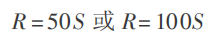这里取 ｔａｎ θ＝1／50 。根据前面的海平面预测数据， 可以预测出海平面上升 对佛罗里达州海岸线的侵蚀量 (见图 １－２１ ) 。显然， 佛罗里达海岸正在受到海水的侵蚀， 虽然被侵蚀的 “速度” 各地不同， 但是海平面的持续上升必然增加大陆遭受海水侵蚀的威胁。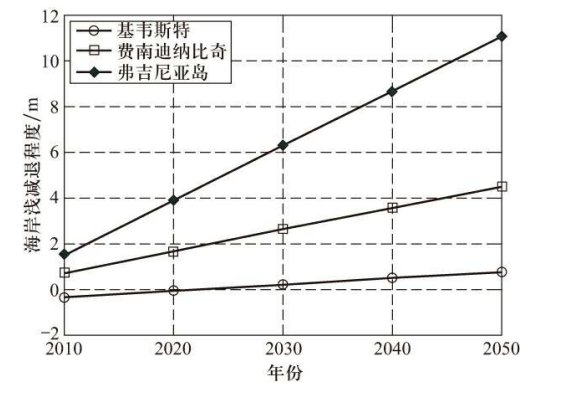图１－２１　佛罗里达附近海平面的上升对海岸线的侵蚀

•  END  •

最新建模竞赛消息扫码关注我们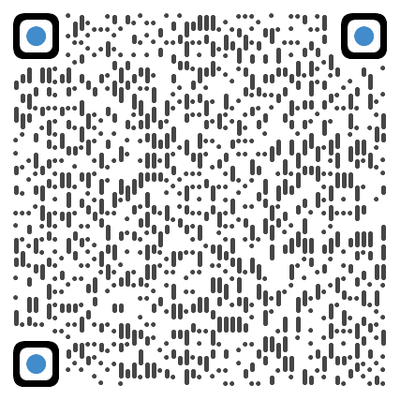2020国际赛QQ参赛群

模友们可能已经发现：现在公众号推送文章的顺序，已经不会按时间排列了。这种变化，可能会让各位模友错过我们每天的推送。

所以，如果你还想像往常一样，聚焦数模乐园，就需要将“数模乐园”标为星标公众号，同时在阅读完文章后，别忘了给一个“在看”哦。

星标步骤

(1)点击页面最上方“数模乐园”，进入公众号主页

(2)点击右上角的小点点，在弹出页面点击“设为星标”，就可以啦。球分享球点赞球在看

展开全文多元相关性分析
• MATLAB多元线性回归: 基于MATLAB的多元非线性回归模型.pdf 多元线性回归建模以及MATLAB和SPSS求解.pdf MATLAB语言在多元线性回归中的应用.pdf
• 多元线性回归模型评估You’ve got a sample dataset and just finished working on a machine learning algorithm using the linear regression model. But now, you are wondering whether or not your analysis ...

多元线性回归模型评估

You’ve got a sample dataset and just finished working on a machine learning algorithm using the linear regression model. But now, you are wondering whether or not your analysis and prediction of the data are accurate, statistically significant, and provides relevant insights needed to solve the problem.

您已经有了一个样本数据集，并使用线性回归模型完成了机器学习算法的研究。 但是现在，您想知道对数据的分析和预测是否准确，具有统计意义，并提供解决问题所需的相关见解。

There are a number of metrics used in evaluating the performance of a linear regression model. They include:

在评估线性回归模型的性能时使用了许多指标。 它们包括：

• R-Squared: seldom used for evaluating model fit

R平方：很少用于评估模型拟合

• MSE (Mean Squared Error): used for evaluating model fit

MSE(均方误差)：用于评估模型拟合

• RMSE (Root Mean Squared Error): always used for evaluating model fit

RMSE(均方根误差)：始终用于评估模型拟合

Let us take a look at each of these metrics, shall we?

让我们看看这些指标中的每一个，对吗？

R-SQUARED:

R平方

• is also known as the coefficient of determination

也称为确定系数
• measures the percentage of variation in the response (dependent) variable explained by the predictor in the predictor (independent) variable.

测量预测变量(独立变量)中预测变量解释的响应(因变量)变化的百分比。
• has values between 0 and 1 for every single regression. Where values between 0.3 and 0.5 refer to a weak r-squared, 0.5 and 0.7 refers to a moderate r-squared, and values > 0.7 refer to a strong r-squared.

每一次回归的值都在0到1之间。 其中介于0.3和0.5之间的值表示弱r平方介于0.5和0.7之间的表示中等r平方，大于0.7的表示强r平方。

• values > 0.7 means that 70% of the variation is around its mean

值> 0.7表示70％的变化均在其平均值附近
• the higher the r-squared, the better the model fits your data (there is a caveat to this…) because there is a possibility of having a low r-squared value for a good model and vice-versa

r平方越高，则模型越适合您的数据(对此有警告)…，因为对于一个好的模型而言，r平方值可能较低，反之亦然

• is a relative measure of model fit. This means they are not a good measure to determine how well a model fits the data.

是模型拟合的相对度量。 这意味着它们并不是确定模型拟合数据的好方法。
• is sometimes considered as statistically insignificant.

有时被认为在统计上微不足道。
• sklearn module : sklearn.metrics.r2_score

sklearn模块： sklearn.metrics. r2_score sklearn.metrics. r2_score

• mathematical formula:

数学公式：

Mean Squared Error (MSE):

均方误差(MSE)：

• measures the average of the squared difference between the observed value and the actual value.

测量观察值与实际值之间平方差的平均值。
• is an absolute measure of model fit.

是模型拟合的绝对度量。
• a value of 0 indicates a perfect fit, this means that the data predict the outcome accurately, however in most cases, it is hardly ever so.

值为0表示完美契合，这意味着数据可以准确地预测结果，但是在大多数情况下，很难做到这一点。
• sklearn module: sklearn.metrics.mean_squared_error

sklearn模块： sklearn.metrics. mean_squared_error sklearn.metrics. mean_squared_error

• mathematical formula:

数学公式：

It is important to understand that

重要的是要了解

Residuals:

残留物：

• is the difference between the actual value and the predicted value

是实际值与预测值之差
• used to check the validity of a model and if assumptions or hypothesis are to be considered

用于检查模型的有效性以及是否要考虑假设或假设
• should be random (i.e has no pattern)

应该是随机的(即没有模式)
• example of a good residual is a scatter plot with residuals centered around 0

良好残差的示例是散点图，残差的中心位于0附近
• statsmodels module: RegressionResults.resid

statsmodels模块： RegressionResults.resid

Root Mean Squared Error (RMSE):

均方根误差(RMSE)：

• is the measure of the distance between the actual values and the predicted value

是实际值与预测值之间的距离的量度
• the lower the RMSE the better the measure of fit. This means that there is little variation in the spread of data

RMSE越低，拟合度越好。 这意味着数据传播几乎没有变化
• is a good measure of how accurately the model predicts the target

是衡量模型预测目标的准确性的好方法
• is considered the best statistics to determine the relationship between the model and the response variable

被认为是确定模型与响应变量之间关系的最佳统计数据
• represents 1-Standard Deviation (residuals) between the actual value and the predicted values

表示实际值和预测值之间的1-标准偏差(残差)
• it measures the spread of the data points from the regression line.

它从回归线测量数据点的分布。
• using sklearn and math module to perform RMSE

使用sklearn和数学模块执行RMSE
• mathematical formula:

数学公式：

It is advisable to have an in-depth knowledge of statistics in order to familiarize yourself with concepts and models used in Data Science. Not sure where to start, this article should give you a headstart into the field of statistics.

建议您具有深入的统计知识，以熟悉数据科学中使用的概念和模型。 不确定从哪里开始， 本文应该为您提供进入统计领域的先机。

It is important to note that these metrics only apply in a regression model and not on a classification model. There are other performance measures that can be employed. I recently worked on a project (red wine quality dataset) and used some of the above metrics to evaluate the performance of my model. Can you tell if this metric performed well or poorly on the problem dataset and why?

重要的是要注意，这些指标仅适用于回归模型，不适用于分类模型。 还有其他可采用的性能指标。 我最近从事一个项目(红酒质量数据集)，并使用上述一些指标来评估我的模型的性能。 您能否说出该指标在问题数据集上的表现好坏，为什么？

Now you know how to work effectively with your dataset using the linear regression model. Thank you for taking the time out to read.

现在，您知道了如何使用线性回归模型有效地使用数据集。 感谢您抽出宝贵的时间阅读。

多元线性回归模型评估

展开全文机器学习 逻辑回归 python 人工智能 深度学习
• 其实上一篇讨论的多元线性回归还不是很全面，而且训练和测试集都没有分，在这里继续讨论多元线性回归模型检验的相关问题。 多元线性回归的输出变量是连续变量，输入变量中如果包含离散变量需要做哑变量或One-hot编码...

https://editor.csdn.net/md/?articleId=105137945
其实上一篇讨论的多元线性回归还不是很全面，而且训练和测试集都没有分，在这里继续讨论多元线性回归模型检验的相关问题。

只要有P值的出现，样本量不超过5000，比如线性回归和逻辑回归；搞清楚算法背后的逻辑才是比较重要的。

多元线性回归需要关注一些什么点？R2和模型稳定性，也就是那些β是不是稳定的，检验模型是不是稳定需要对模型进行诊断。

多元线性回归的输出变量是连续变量，输入变量中如果包含离散变量需要做哑变量或One-hot编码，连续变量可以直接用来建模。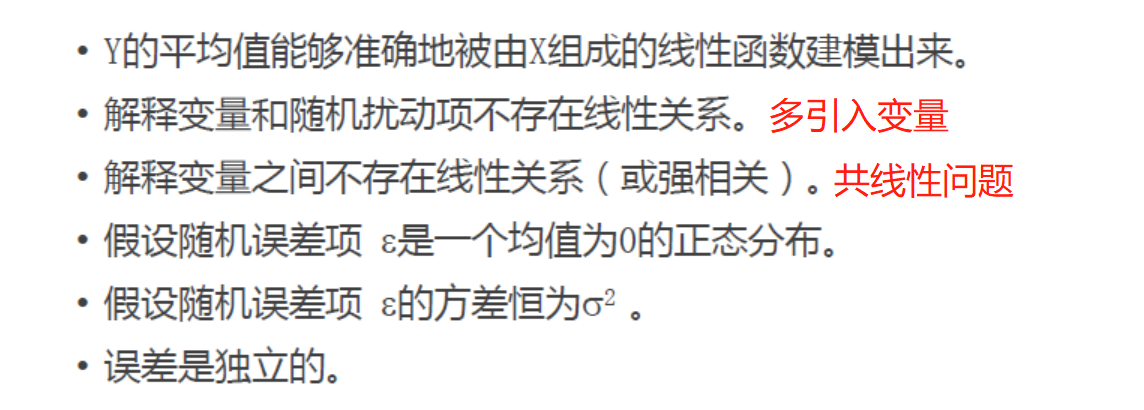多元线性回归假设解释

多元线性回归需要满足的假设其实是比较强的，但是在机器学习或者是数据挖掘领域，后3条针对误差项（其实就是残差）的假设基本上被忽略了。
第1条： 看因变量y和自变量x之间的关系，可以通过绘制散点图，确定是线性、二次函数还是指数函数关系，根据这个来建立x和y之间的关系。后面的神经网络和SVM等模型就是为了方便找到x和y之间的关系。
第2条： 解释变量和随机扰动项不存在线性关系。我们想象一下，如果他们之间存在线性关系的话，是不是会导致回归系数估计的不准确啊，举个例子解释变量y是收入，x是受教育程度，并假设回归方程是 y = 0.5x + e，设想如果扰动项里面包含父母收入，实际上父母收入会影响孩子的收入y，那么回归系数估计值0.5是不是偏高了啊。那怎么解决这个问题呢，那就多纳入一些变量来参与建模吧，这也是多元线性回归存在的必要性，同时这也引出了一个变量筛选的问题。
第3条：解释变量之间不存在线性关系（或强相关）。在建模时，我们不但需要估计回归系数的均值，还需要估计回归系数的标准差：S（β）= S（e）/ |x|，那么如果解释变量x之间存在线性关系的话，分母趋向于0了，回归系数标准差趋于无穷大，所以多重共线性问题是需要去避免的。

多元线性回归诊断方法

如果扰动项是右偏，那么残差图肯定是异方差分布，取对数即可，所以下图中假设5和假设4可以说是一致的。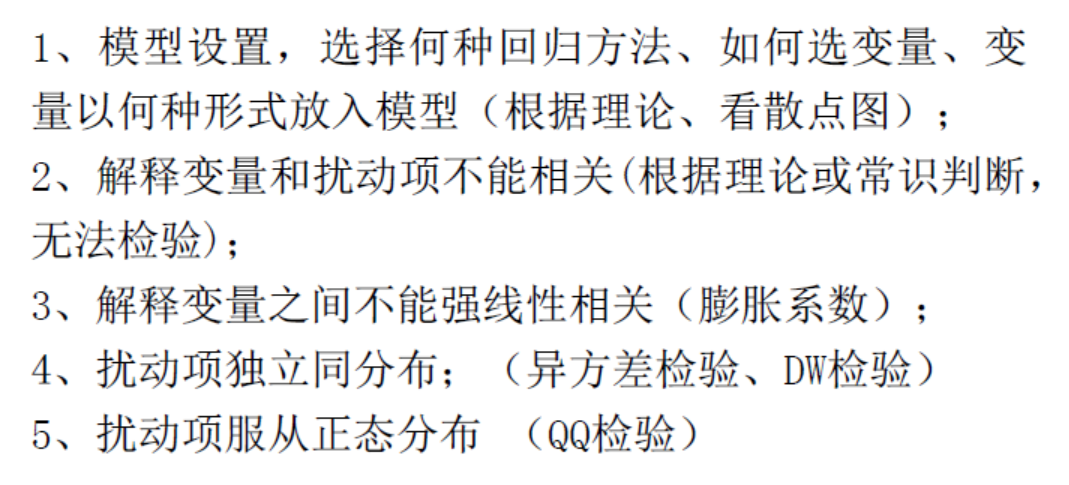多元线性回归模型的诊断

（1）残差分析：实际上当残差不包含任何信息的时候是最好的，如果还包含一些信息，需要把这个信息提取出来。残差图的纵坐标是残差，横坐标可以是各个解释变量x，实际上在做单变量分析，解释变量x被解释变量y做相关性分析的时候就知道了；比如某个解释变量x和被解释变量y都是右偏，那么残差图肯定是异方差，同时取对数重新建模；如果某个解释变量x和被解释变量y存在抛物线关系，那么加入二次项重新进行建模；自相关一般在时间序列数据中比较常见。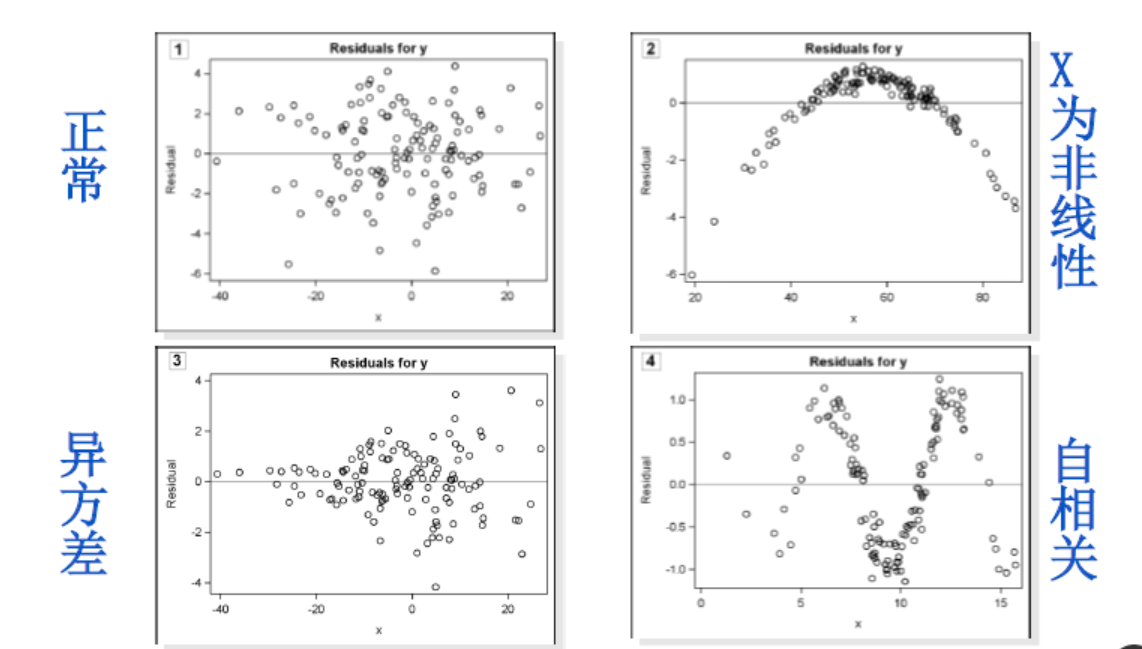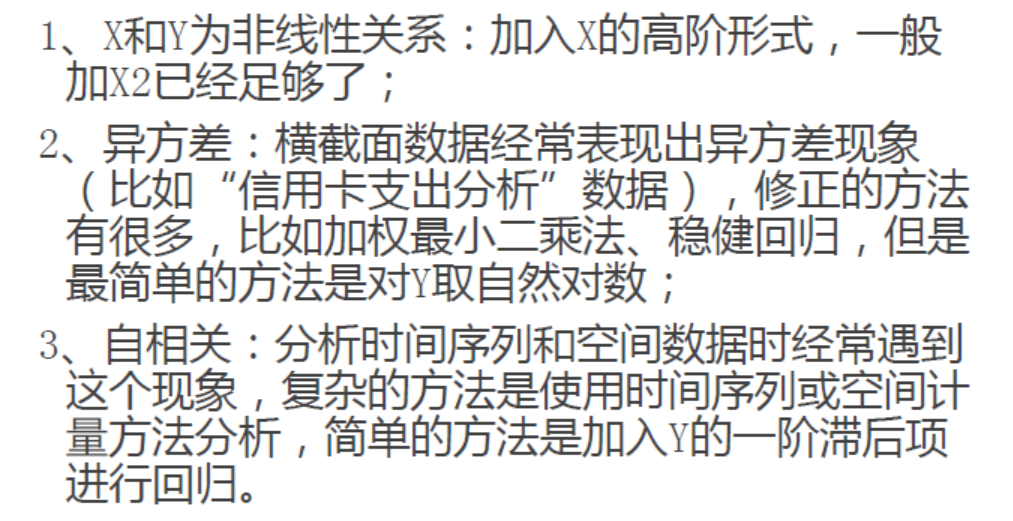（2）强影响点分析
为什么要做强影响点分析？？？因为有了强影响点的存在之后，会把本来没有关系的数据带出关系来，而且这个关系特别不稳定。比如下图，本来数据点之间没有什么关系，但是因为强影响点的存在之后，给数据带出来了这么一个线性关系出来，但这个关系是非常不稳定的，不具有大众性。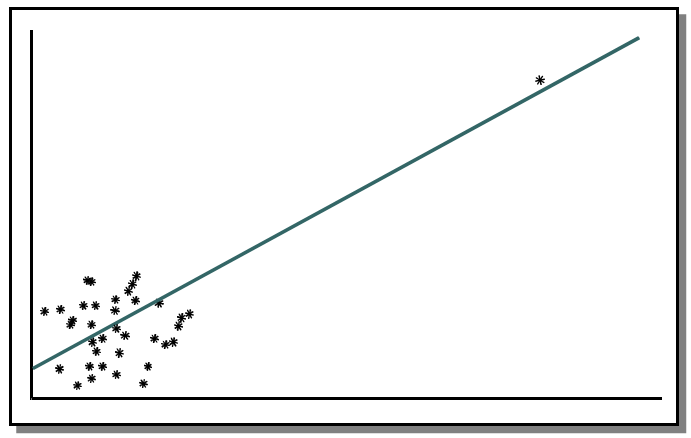怎么解决强影响点分析问题？？？学生化残差（只做一次）。

|SR| = (残差 - 残差均值) / 残差标准差。
|SR| > 2，剔除掉满足条件的记录（几百个样本）
|SR| > 3，剔除掉满足条件的记录（几千个样本）

（3）共线性问题
可以参考下面的链接：https://www.sohu.com/a/326904117_100103806
共线性的判别指标：膨胀系数VIF、相关系数
共线性的解决方法：根据VIF和相关系数手动剔除变量、逐步回归法、岭回归。

展开全文残差分析 多重共线性
• 方差分析三、多元线性回归建模四、模型优化 一、导入包及数据 1. 导入包 import pandas as pd import numpy as np import seaborn as sns import matplotlib.pyplot as plt 2. 导入数据 df = pd.read_csv('house_...线性回归 python 人工智能
• 大家好，我是天空之城，今天给大家带来小福利，带你快速了解一元线性回归方程...多元线性回归模型的系数推导 线性回归模型的假设检验 假设前提 p小于0.3可能不符合线性相关 =============================数据分析 线性回归分析
• 原标题：Python 实战多元线性回归模型，附带原理+代码 作者 | 萝卜来源 | 早起Python( ID：zaoqi-python )「多元线性回归模型」非常常见，是大多数人入门机器学习的第一个案例，尽管如此，里面还是有许多值得学习和...
• 多元线性回归模型检验方法

万次阅读 2019-08-10 22:07:21
终于找到一篇全面而又简洁的讲多元线性回归模型检验方法的文章 PDF下载地址 链接：https://pan.baidu.com/s/1UbyZcMC1VRTmlCEaX4Vybg 提取码：g481 具体内容 一、经济意义检验 经济意义检验主要检验模型参数估计量在...多元回归 F检验 t检验 模型检验
• 点击上方“Python爬虫与数据挖掘”，进行关注回复“书籍”即可获赠Python从入门到进阶共10本电子书今日鸡汤寥落古行宫，宫花寂寞红。前言「多元线性回归模型」非常常见，是大多数人入...机器学习 python 人工智能 数据分析 数据挖掘
• 并通过皮尔森相关性检验、拟合优度检验、F检验、t检验和残差分析的方法对模型进行优化,得到了准确可靠的多元线性回归模型,此楔型具有拟合程度高、简易、直观等优势,为多元线性回归模型在需水量分析中的应用提供了...最小二乘法
• 多元线性回归，主要是研究一个因变量与多个自变量之间的相关关系，跟一元回归原理差不多，区别在于影响因素(自变量)更多些而已，例如：一元线性回归方程 为：毫无疑问，多元线性回归方程应该为：上图中的 x1, x2, xp...
• 多元线性回归模型，假设函数，损失函数，梯度下降及其可视化实现机器学习 多元线性回归
• 多元线性回归模型非常常见，是大多数人入门机器学习的第一个案例，尽管如此，里面还是有许多值得学习和注意的地方。其中多元共线性这个问题将贯穿所有的机器学习模型，所以本文会将原理知识穿插于代码段中，争取以不...python 机器学习 数据分析 数据挖掘
• R语言构建多元线性回归模型 对比一元线性回归，多元线性回归是用来确定2个或2个以上变量间关系的统计分析方法。多元线性回归的基本的分析方法与一元线性回归方法是类似的，我们首先需要对选取多元数据集并定义...人工智能 自然语言处理 机器学习 数据挖掘 R
• 多元线性回归:这是一种线性回归的形式，当有两个或多个预测因子时使用。 我们将看到多个输入变量如何共同影响输出变量，同时还将了解计算与简单LR模型的不同之处。我们还将使用Python构建一个回归模型。 最后，我们...算法 机器学习 人工智能
• 什么是回归分析相关性 ≠ 因果性 ...[外链图片转存失败,源站可能有防盗链机制,建议将图片保存下来直接上传(img-NKvCz36V-1600444325643)(figures/多元线性回归模型/image-20200824222649255.数学建模
• 实验目的：了解R语言多元线性回归模型的建立和检验过程，并能够对其结果结合实际情况进行解释。 实验要求：对给出的两组数据建立线性回归模型，并对实验结果进行分析。 实验数据：1.从给定的数据分析糖尿病人的空腹...r语言
• 回归模型的预测 import matplotlib import matplotlib.pyplot as plt import pandas as pd import numpy as np import seaborn as sns import statsmodels.api as sm from sklearn import model_selection from ...python 机器学习 数据分析
• 多元线性回归模型及stata实现：总论

万次阅读 多人点赞 2020-06-30 20:49:53
多元线性回归方程及stata实现 一、模型 Y=β0+β1X1+β2X2+⋯+βnXn+e Y: Dependent variable（因变量、应变量、反应变量、响应变量、被解释变量等） X1、X2⋯Xn：Independent variable（自变量、解释变量、控制...stata 数理统计
•数学建模
• 3.1多元线性回归模型 一、多元线性回归模型多元线性回归模型的一般形式为： Y=β0+β1*X1+β2*X1+⋯+βk*X1+μ 其中k为解释变量的数目, βj (j=0,1,⋯,k)称为回归系数。上式也被称为总体随机函数的随机表达形式...计量经济学 统计学
• 多元线性回归模型常见问题及解决方法概要多元线性回归模型 基本假设 (1)随机扰动项ui数学期望(均值)为零。E(ui)=0 (2)随机扰动项ui的同方差性且无自相关Var(ui)=σ2 (3)解释变量X列线性无关。R(Xn×k)=K (4)随机扰动...多元线性回归的缺陷
• 小洛写在前面：很多同学目前所做的业务分析工作，徒手分析即可cover业务需求，较少用到一些高阶的统计模型和机器学习上面的东西。渐渐的便会产生一种感觉，即数据分析满足业务需求即可，不需要会...大数据 python 机器学习 人工智能 数据分析
• 线性回归模型属于经典的统计学模型，该模型的应用场景是根据已知的变量（自变量）来预测某个连续的数值变量（因变量）。例如，餐厅根据每天的营业数据（包括菜谱价格、就餐人数、预定人数、特价菜折扣等）预测就餐...
• 实验目的：了解R语言多元线性回归模型中变量的选择和诊断分析 实验要求：对给出的数据用逐步回归的方法选择变量，并对实验结果进行诊断分析。 实验数据：1. 对给出数据进行变量选择，并进行回归诊断。 实验代码： a1...r语言
• 前言：当影响因变量的因素是多个时候，这种一个变量同时与多个变量的回归问题就是多元回归，分为：多元线性回归和多元非线性回归。线性回归(Linear regressions)和逻辑回归(Logistic regressions)是人们学习算法的第...机器学习算法 多元线性回归...